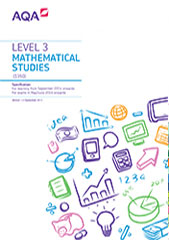# Scheme of assessment

Find past papers and mark schemes, and specimen papers for new courses, on our website at aqa.org.uk/pastpapers

This specification is designed to be taken over two years with all assessments taken at the end of the course.

Level 3 Certificate Mathematical Studies exams and certification for this specification are available for the first time in May/June 2016 and then every May/June for the life of the specification.

This is a linear qualification. In order to achieve the award, students must complete all exams in May/June in a single year. All assessments must be taken in the same series.

All exams in mathematics must include questions that allow students to draw on elements from within and across different topic areas, and questions that allow students to provide extended responses.

All materials are available in English only.

## Aims

This Level 3 Certificate Mathematical Studies qualification will consolidate students' mathematical understanding, build their confidence and competence in applying mathematical techniques to solve a range of problems and introduce them to new techniques and concepts that will prepare them for further study and future employment within a broad range of academic, professional and technical fields.

Mathematical Studies aims to prepare students for the mathematical demands of higher education and work where there is a distinct mathematical or statistical element, but where the mathematical demands do not stretch to a requirement for A-level mathematics.

A course of study leading to this qualification should enable students to:
• study a mathematics curriculum that is integrated with other areas of their study, work or interest leading to the application of mathematics in these areas
• develop mathematical modelling, evaluating and reasoning skills
• solve problems some of which will not be well defined and may not have a unique solution
• solve substantial and real life problems encountered by adults
• use ICT as an exploratory tool for developing mathematical understanding and when solving problems
• develop skills in the communication, selection, use and interpretation of their mathematics
• enjoy mathematics and develop confidence in using mathematics

## Assessment objectives

The assessment objectives (AOs) have been set by AQA under guidance from the Department for Education and Ofqual.

The exams will measure how students have achieved the following assessment objectives.

AO1: Use and apply standard techniques

Students should be able to:

• Accurately recall facts, terminology and definitions
• Accurately carry out set tasks in contexts requiring single- and multi-step solutions

AO2: Select appropriate techniques to solve problems in a mathematical or non-mathematical context and analyse data and represent situations mathematically

Students should be able to:

• Select the mathematics required to solve a problem
• Analyse data and/or given information in order to draw conclusions
• Represent situations mathematically

AO3: Devise strategies to solve problems where the method is not obvious and communicate processes and results

Students should be able to:

• Devise strategies to solve problems where the method is not obvious
• Make realistic assumptions to enable solutions to be obtained
• Evaluate the reasonableness of a solution
• Communicate clearly using appropriate mathematical notation, language and diagrammatic representation

The balance of the assessment objectives may not be equal on each paper.

### Weighting of assessment objectives for Level 3 Certificate Mathematical Studies

Assessment objectives (AOs) Component weightings (approx %) Overall weighting (approx %)
Paper 1 Paper 2
AO1 25-30 25-30 25-30
AO2 31-40 31-40 31-40
AO3 31-40 31-40 31-40
Overall weighting of components 50 50 100

## Assessment weightings

The marks awarded on the papers will be scaled to meet the weighting of the components. Students’ final marks will be calculated by adding together the scaled marks for each component. Grade boundaries will be set using this total scaled mark. The scaling and total scaled marks are shown in the table below.

Component Maximum raw mark Scaling factor Maximum scaled mark
Paper 1 60 x1 60
Paper 2A (Statistical techniques) or Paper 2B (Critical path and risk analysis) or Paper 2C (Graphical techniques) 60 x1 60
Total scaled mark: 120